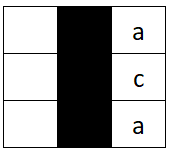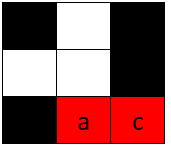Formatted question description: https://leetcode.ca/all/2018.html

# 2018. Check if Word Can Be Placed In Crossword (Medium)

You are given an m x n matrix board, representing the current state of a crossword puzzle. The crossword contains lowercase English letters (from solved words), ' ' to represent any empty cells, and '#' to represent any blocked cells.

A word can be placed horizontally (left to right or right to left) or vertically (top to bottom or bottom to top) in the board if:

• It does not occupy a cell containing the character '#'.
• The cell each letter is placed in must either be ' ' (empty) or match the letter already on the board.
• There must not be any empty cells ' ' or other lowercase letters directly left or right of the word if the word was placed horizontally.
• There must not be any empty cells ' ' or other lowercase letters directly above or below the word if the word was placed vertically.

Given a string word, return true if word can be placed in board, or false otherwise.

Example 1:Input: board = [["#", " ", "#"], [" ", " ", "#"], ["#", "c", " "]], word = "abc"
Output: true
Explanation: The word "abc" can be placed as shown above (top to bottom).


Example 2:Input: board = [[" ", "#", "a"], [" ", "#", "c"], [" ", "#", "a"]], word = "ac"
Output: false
Explanation: It is impossible to place the word because there will always be a space/letter above or below it.

Example 3:Input: board = [["#", " ", "#"], [" ", " ", "#"], ["#", " ", "c"]], word = "ca"
Output: true
Explanation: The word "ca" can be placed as shown above (right to left).


Constraints:

• m == board.length
• n == board[i].length
• 1 <= m * n <= 2 * 105
• board[i][j] will be ' ', '#', or a lowercase English letter.
• 1 <= word.length <= max(m, n)
• word will contain only lowercase English letters.

## Solution 1. Brute Force

Implement a function match(A, s) which returns true if matrix A matches word s horizontally. To handle the vertical case, we can simply use B as the transpose of A, and check match(B, s).

Within match function, we check line by line. For each line, we scan each segment surrounded by #s or line boundaries. For each segment, we check if it equals string s from left to right, or from right to left.

The time complexity is O(MN) because each cell is matched at most 4 times.

// OJ: https://leetcode.com/problems/check-if-word-can-be-placed-in-crossword/
// Time: O(MN)
// Space: O(MN)
class Solution {
bool same(vector<char> &A, int first, int last, string &s) { // returns true if A[first..last] equals s or reversed s.
if (last - first + 1 != s.size()) return false;
int i = 0, N = s.size();
while (i < N && (A[first + i] == ' ' || A[first + i] == s[i])) ++i; // match from left to right
if (i == N) return true;
for (i = 0; i < N && (A[last - i] == ' ' || A[last - i] == s[i]);) ++i; // match from right to left
return i == N;
}
bool match(vector<vector<char>> &A, string s) { // returns true if matrix A matches string s horizontally
int N = A.size();
for (auto &row : A) {
for (int i = 0; i < N; ) {
while (i < N && row[i] == '#') ++i;
int start = i;
while (i < N && row[i] != '#') ++i;
if (same(row, start, i - 1, s)) return true; // match row[start..(i-1)] with s.
}
}
return false;
}
public:
bool placeWordInCrossword(vector<vector<char>>& A, string s) {
int M = A.size(), N = A.size();
vector<vector<char>> B(N, vector<char>(M)); // B is the transpose of A
for (int i = 0; i < M; ++i) {
for (int j = 0; j < N; ++ j) {
B[j][i] = A[i][j];
}
}
return match(A, s) || match(B, s);
}
};